1

# Reorder edges

Difficulty: MEDIUM
Avg. time to solve
30 min
Success Rate
70%

Problem Statement
Suggest Edit

#### Input Format

``````The first line of input contains an integer 'T' representing the number of test cases.

The first line of each test case contains a single integer, ‘N’, where ‘N’ denotes the total number of nodes in the given graph.

The next ‘N’ - 1 lines contains two space-separated integers, ‘U’ and ‘V’, where ‘U’ and ‘V’ represent the nodes that share a directed edge from ‘U’ to ‘V’.
``````

#### Output Format:

``````For each test case, print the minimum number of operations required to make a directed path from each node to node 0.
The output of each test case will be printed in a separate line.
``````

#### Constraints:

``````1 <= T <= 5
1 <= N <= 3000
0 <= U, V <= N - 1

Where ‘T’ is the number of test cases,  ‘N’ denotes the total number of nodes in the given graph, and ‘U’ and ‘V’ denotes the nodes that share a common directed edge between them.
``````

#### Note:

`````` You do not need to print anything, it has already been taken care of. Just implement the given function.
``````

#### Sample Input 1:

``````2
4
0 1
0 2
1 3
5
1 0
2 0
3 1
4 1
``````

#### Sample Output 1:

``````3
0
``````

#### Explanation of Sample Input 1:

``````Test Case 1 :
The given graph is shown below.
``````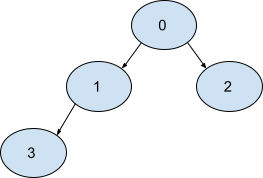``````We can reverse edge 0 -> 1, 1 -> 3 and 0 -> 2. The resulting graph will be:
``````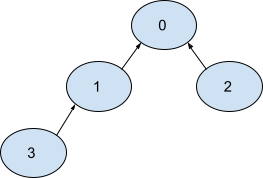``````Now there exists a directed path from 1, 2, and 3 to 0 i.e., 1 -> 0, 2 -> 0, and 3 -> 1 -> 0 respectively.
So the minimum number of operations required will be 3.

Test Case 2 :
The given graph is shown below.
``````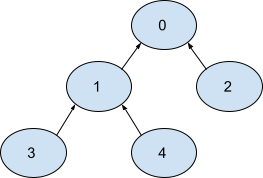``````There is already a directed path from nodes 1, 2, 3, and 4 to 0 i.e., 1 -> 0, 2 -> 0, 3 -> 1 -> 0, and 4 -> 1 -> 0 respectively.
So the minimum number of operations required will be 0.
``````

#### Sample Input 2:

``````1
5
1 0
2 0
1 3
2 4
``````

#### Sample Output 2:

``````2
``````

#### Explanation of Sample Input 2:

``````Test Case 1 :
The given graph is shown below.
``````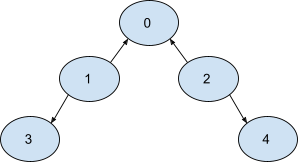``````We can reverse edge 1 -> 3,  and 2 -> 4. The resulting graph will be:
``````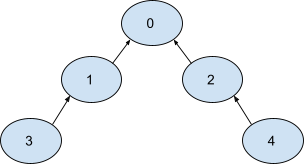``````Now there exists a directed path from 1, 2, 3 and 4 to 0 i.e., 1 -> 0, 2 -> 0, 3 -> 1 -> 0, and 4 ->. 2 -> 0 respectively.
So the minimum number of operations required will be 2.
``````Want to solve this problem? Login now to get access to solve the problems